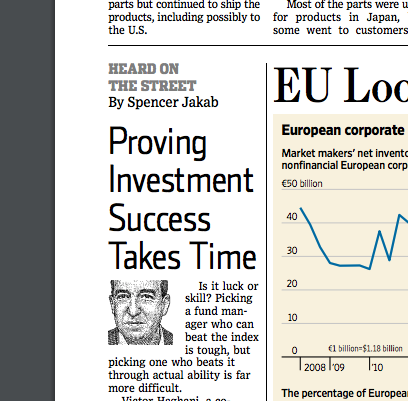# Making sense of a probability problem in the WSJSomeone wrote to me the other day asking if I could explain a probability example from the Wall Street Journal. (“Proving Investment Success Takes Time,” Spencer Jakab, November 25, 2017.)

Victor Haghani … and two colleagues told several hundred acquaintances who worked in finance that they would flip two coins, one that was normal and the other that was weighted so it came up heads 60% of the time. They asked the people how many flips it would take them to figure out, with a 95% confidence level, which one was the 60% coin. Told to give a “quick guess,” nearly a third said fewer than 10 flips, while the median response was 40. The correct answer is 143.

The anecdote is correct in spirit: it takes longer to discover the better of two options than most people suppose. But it’s jarring to read the answer is precisely 143 when the question hasn’t been stated clearly.

How many flips would it take to figure out which coin is better with a 95% confidence level? For starters, the answer would have to be a distribution, not a single number. You might quickly come to the right conclusion. You might quickly come to the wrong conclusion. You might flip coins for a long time and never come to a conclusion. Maybe there is a way a formulating the problem so that so that the expected value of the distribution is 143.

How are you to go about flipping the coins? Do you flip both of them, or just flip one coin? For example, you might flip both coins until you are confident that one is better, and conclude that the better one is the one that was designed to come up heads 60% of the time. Or you could just flip one of them and test the hypothesis Prob(heads) = 0.5 versus the alternative Prob(heads) = 0.6. Or maybe you flip one coin two times for every one time you flip the other. Etc.

What do you mean by “95% confidence level”? Is this a frequentist confidence interval? And do you compute the (Bayesian) predictive probability of arriving at such a confidence level? Are you computing the (Bayesian) posterior model probabilities of two models, one in which the first coin has probability of heads 0.5 and the second has probability 0.6 versus the opposite model?

Do you assume that you know a priori that one coin has probability of heads 0.5 and the other 0.6, or do you not assume this and just want to find the coin with higher probability of heads, and evaluate such a model when in fact the probabilities of heads are as stated?

Are you conducting an experiment with a predetermined sample size of 143? Or are you continuous monitoring the data, stopping when you reach your conclusion?

I leave it as an exercise to the reader to implement the various alternatives suggested above and see whether one of them produces 143 as a result. (I did a back-of-the-envelope calculation that suggests there is one.) So the first question is to reverse engineer which problem statement the article was based on. The second question is to decide which problem formulation you believe would be most appropriate in the context of the article.

## 6 thoughts on “Making sense of a probability problem in the WSJ”

1. I’ve been using examples like this for my basic introduction to probability and estimation. I was inspired by the intro to Jim Albert’s great baseball stats book Curve Ball.

I’m not enough of an applied mathematician to do this one back of the envelope! A quick simulation shows that 143 draws from bernoulli(0.6) yield a roughly 85% chance of rejecting the null hypothesis that the chance of success is less than or equal to 0.5. A null hypothesis that the chance of success is equal to 0.5 leads to a roughly 70% rejection rate. This only assumes we throw the 0.6 coin and only know there’s a 0.5 coin and another coin.

Here’s some R code (rbinom is simulating, pbinom is the CDF)

> for (n in 1:1000) y[n] sum(y > 0.95) / 1000
 0.83

> sum(y > 0.975) / 1000
 0.72

What do you think the best way to visualize this is? Density plots of the maximum likelihood estimators (sampling rbinom(N, 143, 0.5) / 143 and rbinom(N, 143, 0.6) / 143) is one way to go—it’s easy to see their tails overlap considerably. I wish someone had plotted such distributions of estimators when I was trying to learn math stats the first time!

2. Is there some kind of markdown this comment system supports?

I’ll try to escape the comparison operators this time and not use <pre> or <code>

> for (n in 1:1000) y[n] <- pbinom(rbinom(1, 143, 0.6), 143, 0.5)

> sum(y > 0.95) / 1000
 0.83

> > sum(y > 0.975) / 1000
 0.72

3. You can use most HTML tags for markup. It’s a little particular about code vs pre. The former is for inline use and the latter for blocks. The most common problem I see is people using code for a block.

4. Bill Raynor

If all you want is the largest one with probability .95, rather than a significant one, the common sample size is 60, according to table E1 of “Selecting and Ordering Populations” by Gibbons et.al.

The procedure is to make the 60 tosses for each coin and pick the one with the most successes.